# How to find the minimum value in range while ignoring 0 value in Microsoft Excel

To find the minimum numerical value in range while ignoring the 0 value, we can use the Small function along with Countif function in Microsoft Excel.

SMALL: - This function is used to return the kth smallest value in a data set.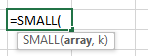Example: Column A contains a list of numbers from which we need to find out the  smallest number.

• Select the cell B1.
• Write the formula =SMALL(A1:A4,1)
• Press Enter on your keyboard.
• The function will return the smallest number in the row.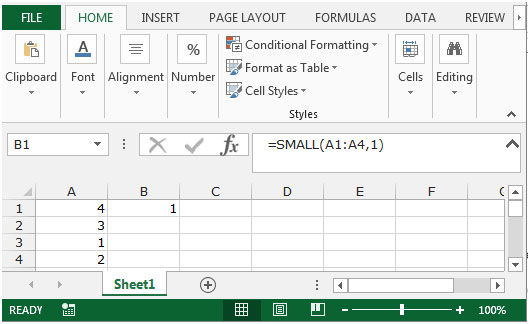COUNTIF:  This function is used to count the number of cells within a range which meet a specified condition.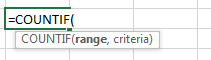For Example:- We have data in range A2:A11 in which we need to count how many times the states are repeating in Column A.

• Select the cell B2, and write the formula =Countif (A2:A11, A2) press Enter on the keyboard.
• The COUNTIF function will return 4 which means that “Washington” is repeating 4 times in Column A.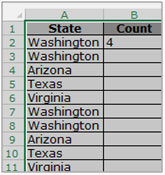In this article,lets use the Small function to find out the smallest value in the range and the CountIf function will help us toignore the 0 value while choosing the smallest number from the range.

Let’s take an example to understand how we can find the minimum numerical value in a range while ignoring the 0 value.

We have data for items in range A1:C11. Column A contains Zone, column B contains Agent name and column C contains sold unit.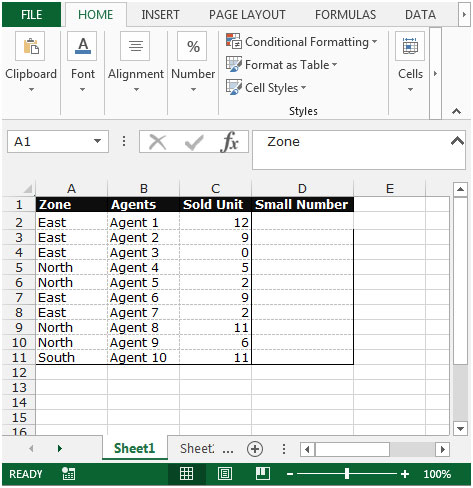If you want to find the minimum number of sold quantity to ignore the 0, follow the below given steps:-

• Select the cell D2.
• Write the formula to find out the small value
• =SMALL(C1:C11,COUNTIF(C1:C11,0)+1)
• Press Enter on your keyboard.
• The function will return the minimum value in the range while ignoring the 0 value.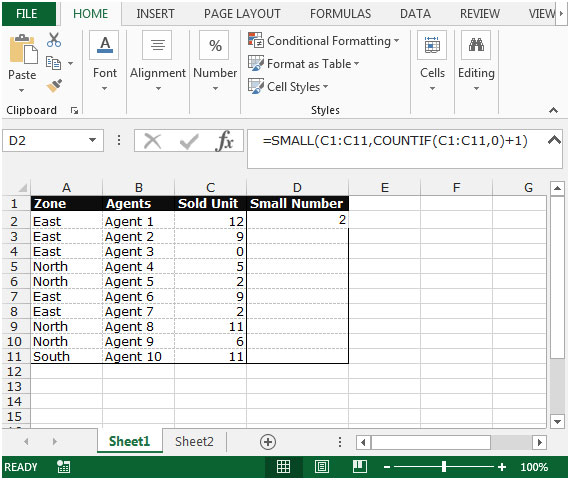This is the way we can find out the minimum number in a range while ignoring 0 value by using the Small function along with the COUNTIF function in Microsoft Excel.We would love to hear from you, do let us know how we can improve, complement or innovate our work and make it better for you. Write us at info@exceltip.com

1.I use the formular but it still me zero as the smallest

2."more ""universal"" way to achieve the same result will be
{=min(if(A1:A7=0,max(A1:A7),A1:A7)}

(brackets {} means that you should press Ctrl-Shift-Enter to complete the formula)"

Terms and Conditions of use

The applications/code on this site are distributed as is and without warranties or liability. In no event shall the owner of the copyrights, or the authors of the applications/code be liable for any loss of profit, any problems or any damage resulting from the use or evaluation of the applications/code.